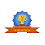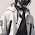# vs.eyeandcontacts.com

## Chapter 1 Number System

Brain Developers
A. Fill in the blanks:
1. The base of Binary number system is
2.
2. The base of
Decimal number system is 10.
3. Octal number system consists of
8 digits.
4. In Binary addition, 1 + 1 equals to
10.
5.
Binary number system is understood by the computer system.
6.
Hexadecimal uses 16 symbols to represent numbers.
7. In Binary subtraction, 1 - 1 equals
0.

B. State True or False:
1. The decimal number system consists of 10 digit i.e., 0 to 9. (
True)
2. The method to perform division of two binary number is not same as that of the decimal numbers. (
False)
Correct Answer: The method to perform division of two binary number is same as that of the decimal numbers.
3. 1 multiplied by 0 equals to 0. (
True)
4. Charles Babbage introduced the concept of 0 [Zero]. (
False)
Correct Answer: Aryabhatt introduced the concept of 0 [Zero].
5. The number used in Octal number system are 1 to 7. (
False)
Correct Answer: The number used in the Octal number system are 0 to 7.

C. Application Based Question:
1. Ratika's computer teacher asked her to convert the Octal number to Decimal number. Suggest her the method which she should apply in converting the Octal number.
Multiply each octal number with 8 having the power 0 for unitary position,starting from the extreme right digit.

2. The teacher has given as assignment to Saurabh on Binary subtraction. Saurabh is confused how to subtract 1 from 0. Help him solving the problem.
He can solve this by borrow method as (0 - 1 = 1).

D. Multiple Choice Questions:
1. ____________ introduced the concept of 0 [Zero].
b) Aryabhatt
c) Bill Gates
b) Aryabhat

2. A _________ converts the decimal format into its binary equivalent.
a) Digital computer
b) Cell phone
c) Abacus
a) Digital Computer

3. A computer understands only _________ code.
a) English
b) French
c) Binary
c) Binary

4. In Binary multiplication, 1 x 1 equals to _______.
a) 0
b) 1
c) 2
b) 1

5. To convert Decimal number into Binary number, divide the number by ________.
a) 2
b) 8
c) 10
a) 2

1. Explain Number system and its commonly used types.
Number system is a way to represent the numbers in the computer architecture. There are four different types of number system -
a) Binary number system
b) Octal number system
c) Decimal number system

2. What are the rules to convert a Decimal number into Binary number?

Step 1: Divide the given decimal number with the base 2.
Step 2: Write down the remainder and divide the quotient again.
Step 3: Repeat the step 2 until the quotient is 0.

3. Write the rules to multiply 2 binary numbers.
The rule for performing multiplication using binary numbers is the same that of the numbers. 0 x 0 = 0, 0 x 1 = 0, 1 x 0 = 0 & 1 x 1 = 1.

4. Briefly explain the Octal number system.
Octal number system consists of 8 digits i.e . 0 to 7 with the base 8.

5. What do you understand by hexadecimal number system?
Hexadecimal number system contains 16 digits, and therefore, has the base 16. It uses the digits (0 - 9) and (A - F).

1.thanks for this amazing solution but please mention Que-5 what do you under stand by hexadecimal number system? in this solution please and thanks

1.2.Keep visiting our website!

3.4.5.nice i had a good practice of my concepts for my exam

6.Thanks! In this website you can also find NCERT Solutions... Do recommend this website to your friends if you found the solutions helpful!

7.Thank you very much

8.Welcome! Do share our website with your friends!

2.please make notes for this chapter

1.Ok sure! We shall consider your suggestion...

2.3.The question is 👇🏻
What do you understand by the Hexadecimal number system?

4.The Question is 👇🏻
What do you understand by the Hexadecimal number system?

5.Okay, thanks for updating us! We have added Question 5, do check once...

3.4.Good One Sir...Put the Textbook Sums as well..

1.Ok sure! We shall...

5.Anonymous12 July, 2021

Thanks for this post

1.Welcome :)

6.Anonymous13 July, 2021

1.Thanks for your positive feedback! 😀

7.NICE SUPER USEFUL OF THIS SOLUTIONS I LIKE IT IT WAS EAST

1.8.1.2.5th question is "what do you understand by hexadecimal number system?"

3.Hi user,

This number system contains 16 digits, and therefore, has the base 16. It uses the digits (0 - 9) and (A - F).

9.1.Could you please type that question, so that we can get back to you...

10.1.Please check once again... It is written step 2 and not step 3

11.12.thank you sir

1.2.3.Welcome! It is our pleasure to share our knowledge with you... Thanks to you too!

13.14.good i love it very help me

1.Welcome! Do recommend our website to your friends if you found our website helpful...

2.3.Hi,
Actually we are providing solutions of Kips IT Beans Class 6 and Class 7 only.

15.Thanks
Sir

1.Thanks to you too. Keep visiting our website! For the development of this site please click atleast one ad so that we have improve our resources to make it more useful for students...

16.Please I want the notes of the chapter number system because I think it is a bit tough

1.Okay sure! We shall post it... When we will post it, we will let you know... Subscribe to our website for latest update.

17.Please post the pdf of book also

1.Okay Aman Sharma! Thanks for your feedback...

18.Yes it's brilliant wow nice 😄👍

1.Thanks! Do share our website with your friends...

19.Thank you so much

1.Welcome! You can also find solutions to Kips IT Beans, Cyber Apps, Cyber Beans, Artificial Intelligence...

HomeTop